Feb 13, 2015
55725 Views

# Grade 2 Maths Complete Worksheets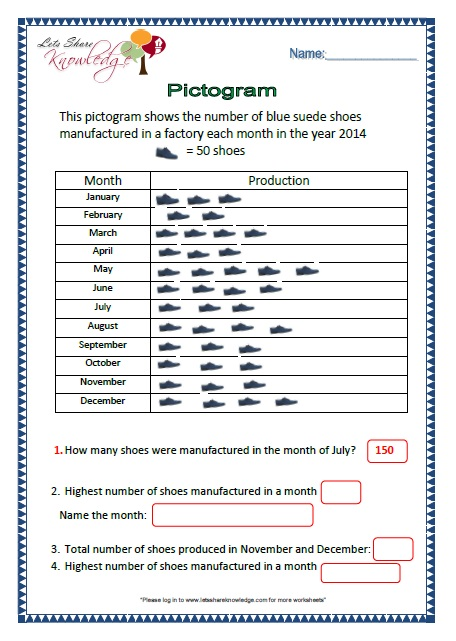Maths Grade 2 Topics and Worksheets

# 4 – Digit Numbers

Thousands, Hundreds, Tens and Units – 4 Digits (Blocks):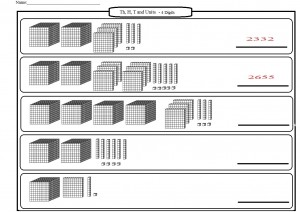Thousands, Hundreds, Tens and Units.
Placing value of numbers to 9999.

Reading and Writing Numbers – 4 Digits: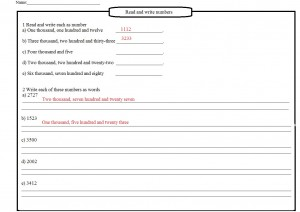Set 1 – Writing from words to numbers and from numbers to words. Click here for Set 1Set 2 – Circle the correct digit and write the value of given digit. Click here for Set 2

Using an Abacus for 4 Digits:

Writing the numbers shown on each abacus.
Draw the correct number of beads to match the number written below the abacus.
Making numbers using the given bead.Comparing Numbers:

Comparing Numbers using sign “<” or “>”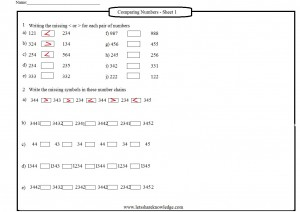# 4 – Digit Numbers

Using an Abacus for 5 Digit Numbers

Writing numbers shown on abacus and how to place beads on an abacus to represent numbers.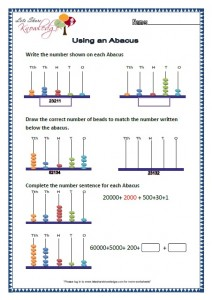Rounding to the nearest 10 and 100:

Rounding off numbers to nearest 10 for example 183 would be 180 and rounding off to the nearest 100 where 183 would be 200.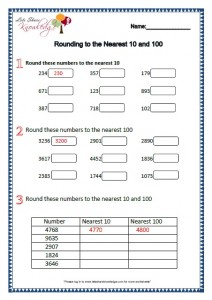Reading and Writing Numbers 5 Digits:

Reading and writing numbers in words.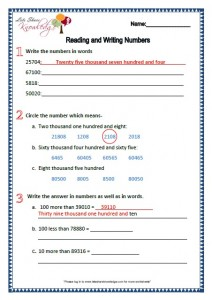Putting Numbers in Order

Putting the numbers smallest to largest
For example 197, 134, 143 would be 134, 143 and 197. Then largest to smallest for the same question would be 197, 143 and 134.Place Value: 5 Digit Numbers:
Placing values of 5 digit numbers. Like in 23467 the number 3’s value would be 3 thousand.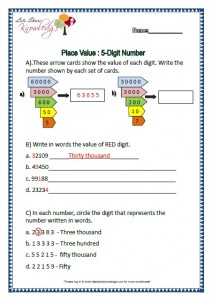Renaming of number means using Units, Tens Hundreds, Thousands and so on for addition purpose.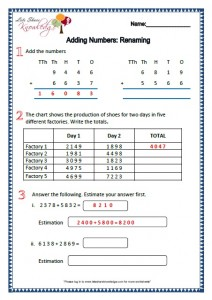No renaming means not using Units, Tens, Hundreds and so on for addition.Adding 4 Digit Numbers: No Renaming

No renaming means not using Units, Tens, Hundreds and so on for addition.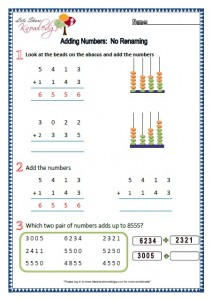2341 + 2222 + 1221 = 5784.# Subtraction

Subtracting 4 Digit Numbers: No Renaming:
Crossing out beads on the given abacus, subtracting the numbers and writing the difference between the given two numbers.Click here
Subtracting 3 Digits Numbers:
With Exchange:
Subtracting the given numbers,
Writing which two numbers have a differnce of the given number in question and filling in the blanks with numbers 1, 2, 3, 4 or 5.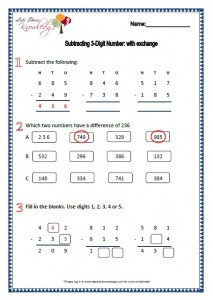Click here
Subtracting 4 Digits Numbers: Renaming:
Subtracting the given numbers, solving the problems and writing the difference of given numbers.Click here

# Properties and Patterns of Numbers Worksheets

Number Properties and Pattern: Writing the numbers given on the scale which are ointed by an arrow, writing the missing numbers in sequence and writing the numbers which are halfway between the two numbers.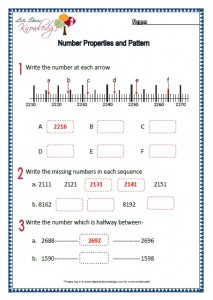Counting 10’s, 100’s and 1000’s: Looking at the abacus and stating the addition or subtractions of 10’s, 100’s or 1000’s, then writng the next three numbers in a given pattern and lastly writing the missing numbers based on a sequence.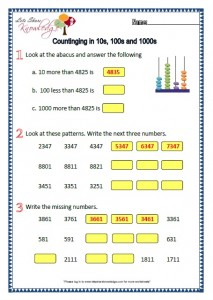Even and Odd: Sorting out the even and odd numbers. Circling the smallest or biggest even or odd numbers and colouring the blocks yellow having most of either even or odd.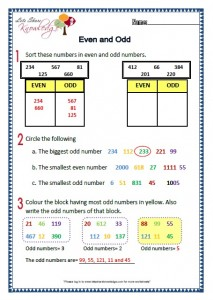Number Pattern: Writing the next two numbers in the given number pattern.Writing the missing numbers in each sequences and making a 5 number sequence.Patterns and Problems: Counting in groups and writing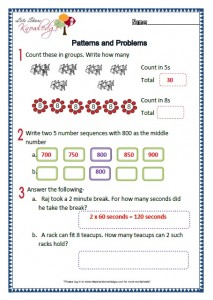# Time Worksheets

Time: Writing the months in order, completing questions and choosing the correct answers based on minutes, hours, days, weeks, months and years.Minutes Past Hour: Writing the time on the clocks as minutes past the hour. Drawing the given time on the clock face and showing the time on the clock faces.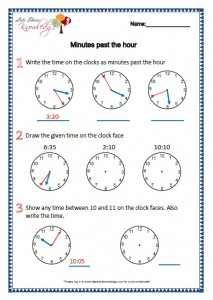Reading the Time: Write the time on the clock faces in numbers and in words. Write the time given on the clock faces. What is the difference in time? Time problems.Calendars: Answering questions by looking at the Calendars.Time Problems: Writing timings, answering questions based on time schedule given and solving problems based on time.# Multiplication Worksheets

Multiplication Facts: Random questions based on multiplication.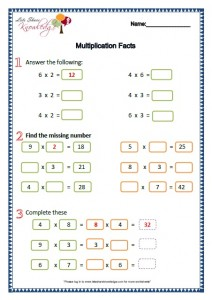Multiplying by 9: Practice questions on multiplication by 9.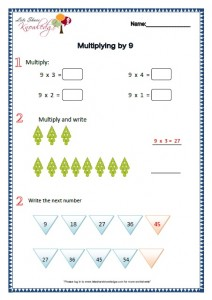Multiplying by 6: Practice questions on multiplication by 6.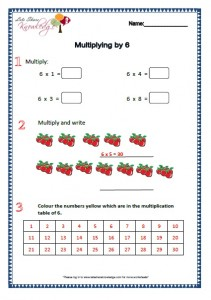Multiplying by 10 and 100: Practice questions on multiplication by 10 and 100.Multiplying by 7: Practice questions on multiplication by 7.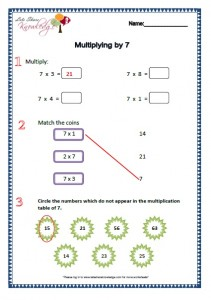Multiplying Multiples of 10: Complete questions like 5 * 10 = 50, and word problems.Multiplying by 8: Practice questions on multiplication by 8.TU * U No carrying: Multiplication sums and Filling in the correct signs ( <, =, >).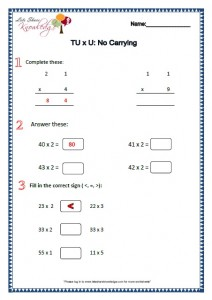TU * U with carrying: Multiplication and using different digits to get answers.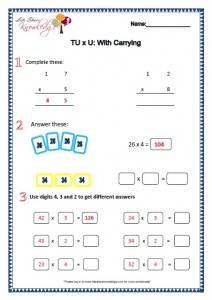# Division Worksheets

Division: Grouping: Grouping into numbers given and word problems.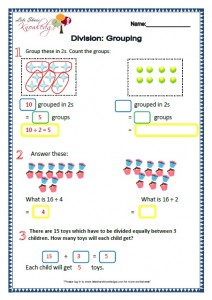Numberlines: Using numberlines to answer questions.Division: Repeated Subtraction: Solving questions based on division with repeated subtraction.Division without remainder: Division sums without remainder like 10 divided by 5 equals 2 and few word problems.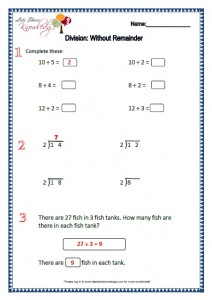Division and Multiplication: Questions based on multiplication and division.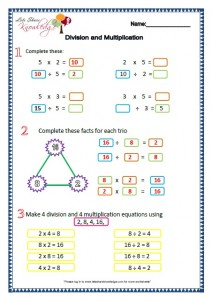# Angles

Angles and Turnings: Writing directions in which the arrow will turn, clockwise or anti clockwise.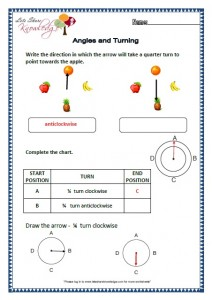Right Angles: Questions based on understanding right angles.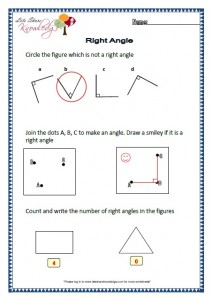Types of Angle: Writing the names of angles formed, telling what angle is the birdie’s beak showing and drawing line segments.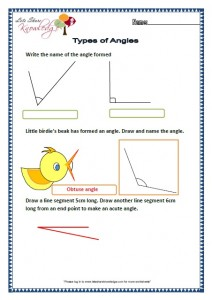Angles and Shapes: Writing the names of angles formed, telling what angle is the birdie’s beak showing and drawing line segments.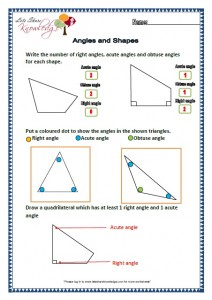# Symmetry

Symmetry: These worksheets are about drawing the reflection of each shape, drawing lines of symmetry and circling the alphabets where the line of symmetry cannot and where it can be drawn.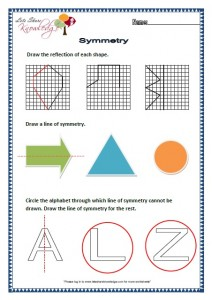Symmetrical Shapes: These worksheets are about drawing another line of symmetry, drawing two lines of symmetry and telling Which of the shapes do not have at least 2 lines of symmetry? Circle it. Draw 2 lines of symmetry for the other.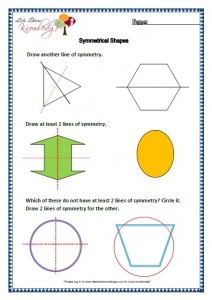# Shapes

Squares and Rectangles: These worksheets are about drawing squares and rectangles.Triangles: These worksheets are based on different kinds of triangles, like isosceles, right angled etc.Drawing Shapes: These worksheets are about drawing shapes based on given measurements.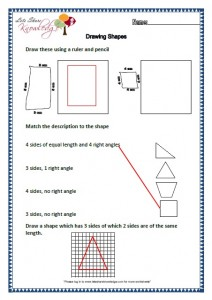Naming Solid Shapes: These worksheets are about naming solid shapes like cone, cube, sphere etc.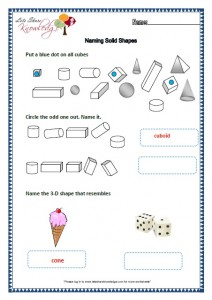Faces of Shapes: These worksheets are about identify how many faces does a shape has and questions related.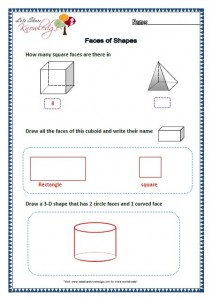Properties of Shapes: These worksheets are about properties of shapes like knowing about an edge, side or a face.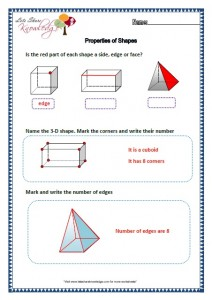Nets of Shapes: Naming the 3D shapes, colouring squares to complete the net of 3D shapes and answering if the given diagram is a net of a 3D shape.# Functions

Inverse Relations Addition and Subtraction: Completing the equations and making addition and subtraction equations.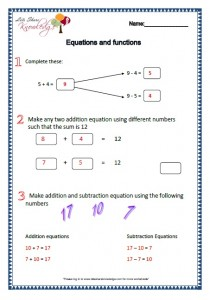Equation and Equalities: Using the correct signs (<,=,>), putting numbers in the box to correct the statement and filling in the given numbers to complete the equations.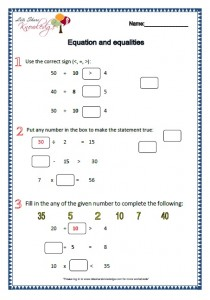Inverse Relation Multiply and Divide: Basic Multiplication and Division questions.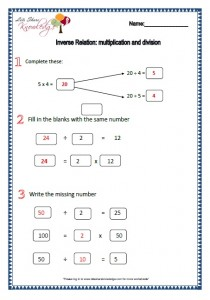Missing Numbers: Completing the missing numbers. Solving the equations.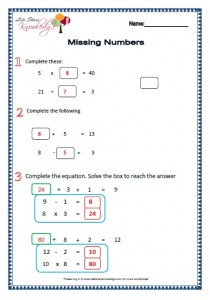# Measures

Measuring Length: Using the correct sign (<,=,>), converting like from meters to centimetersEstimating Length and body units: Measuring lengths using hand spans, converting measurement to centimeters and comparing the found out measurements.Measuring Weights: Using the correct signs, converting like kg to grams and matching the given  numbers.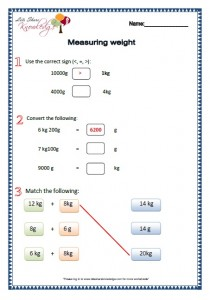Measuring Capacity: Converting like litres to millilitres, choosing the correct sign (<,=,>) and matching.Measuring Problems: Writing the total weight, questions on how much ml required and solving problems.# Perimeter and Area

Perimeter of Shapes: Finding the Perimeters of the shapes given. Drawing the shapes based on the measurements given. Answering which of the shapes have greater parameter.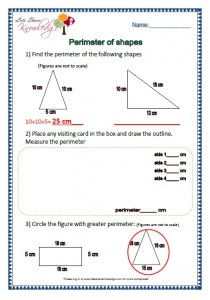Perimeter of Rectangles and Squares: Finding the perimeters of the rectangles and squares. Colouring the parameter of the shapes that is asked.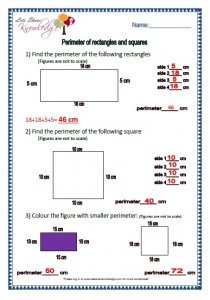Areas of Shapes: Finding the areas of the shapes given. Colouring to make areas as asked and writing the approximate areas of the figures.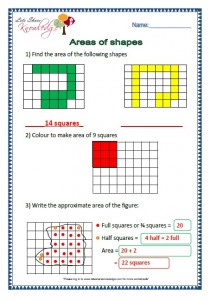Area of Rectangles: Finding the areas of the rectangles given. Coloring the areas as asked, and finding the areas of the given measurements.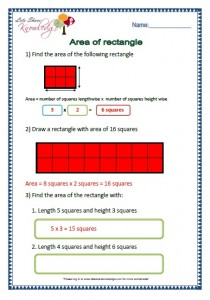# Fractions

Numerators and Denominators: Writing the fractions. Answering what fractions are coloured and colouring the fractions asked.Fractions on Number Lines: Answering what fraction is the number line showing. Marking the fraction given.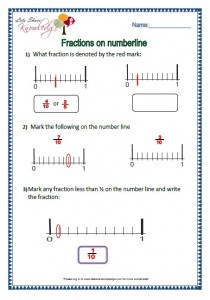Comparing Like Fractions: Writing the same fractions. Crossing the smallest and circling the biggest fraction. Drawing and colouring a like fraction.Fractions of Numbers: Circling based on fraction asked. Solving fractions and answering problems.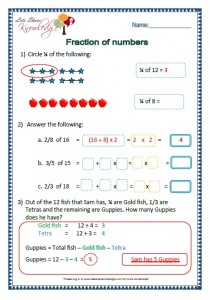Fractions and Money: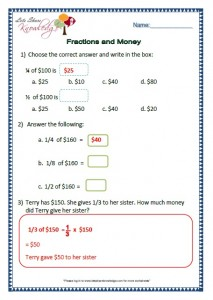# Handling Data

Pictograms: Answer questions based on the pictogram given.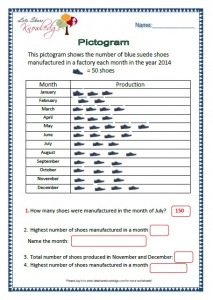Bar Graphs Different Scales: Giving answers of the questions based on the bar graph given using different scales.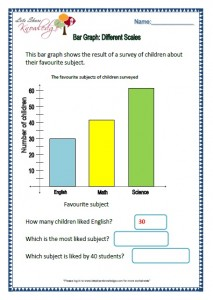Bar Graphs: Giving answers to questions based on bar graphs.Probability: To find if the event is likely, unlikely, certain or impossible. Circling the correct answers and drawing based on the probability asked.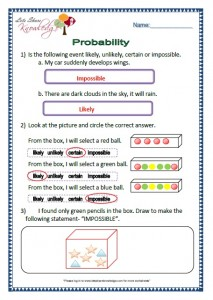Somaira Khatri January 6, 2018 3:15 pm Reply